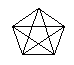Email us to get an instant 20% discount on highly effective K-12 Math & English kwizNET Programs!

#### Online Quiz (WorksheetABCD)

Questions Per Quiz = 2 4 6 8 10

### MEAP Preparation - Grade 4 Mathematics3.10 Diagonals

 A diagonal is a line segment that is not a side and that joins two vertices of a polygon. Directions: Answer the following. Also draw a triangle, square and rectangle and show its diagonals.
 Q 1: From the figure can you find out the number of diagonals for a 10 sided polygon?373527 Q 2: The figure gives the number of sides and their diagonals. What is the pattern?2,4,5,63,4,5,6,71,2,3,4 Q 3: A hexagon has ______diagonals.698 Q 4: If the diameter of a circle is 28 meters, what is the radius?28 meters2 meters85 meters14 meters Q 5: A pentagon has ______diagonals.4567 Q 6: A diagonal is a line segment that is not a side and that joins two vertices of a polygon.falsetrue Question 7: This question is available to subscribers only! Question 8: This question is available to subscribers only!